# FAQ

## Acquisition

You can add our add-in through Microsoft Appsource store. To visit the store, please open Excel, click on Insert and on Add-ins. Here you can search ‘Erlang’ and add the add-in to your Excel. The add-in can be found under Home at the far right of the ribbon.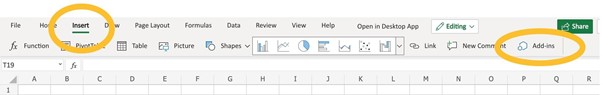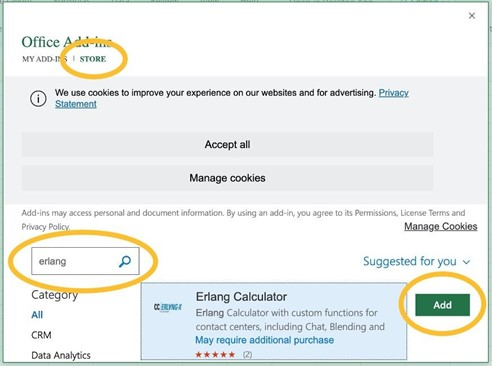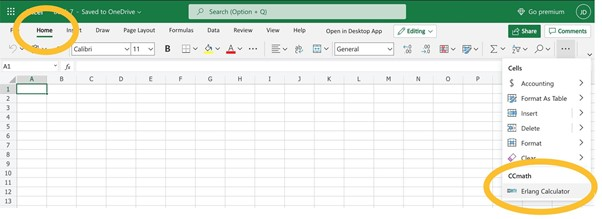## Error Messages

Please sign in with your Microsoft 365 account. You can sign in by opening the add-in under Home, and click on ‘Sign in with Microsoft’ in Excel.When this error message appears, you have used an invalid parameter. To find out which of the parameters is invalid, please read the manual carefully. Here you can find definitions of the parameters and functions, and which parameters must or may be used in the function.
This error message may appear when you are logged in, but your session is expired. Please sign out of the add-in in Excel, and sign in again. This will start a new session and you will be able to use the functions again.

## Examples

The Erlang C model is defined as a queuing system where customers arrive to the system according to a Poisson process and are served by a fixed number of servers. Customers that find all servers busy are queued in a queue with infinite buffer capacity. The service times are exponentially distributed. This model uses the following input parameters from the historical data:

• Call arrivals per minute (Forecast-FC)
• Average Handling time in minutes (AHT)
• Acceptable Waiting Time in minutes (AWT)
• Number of Agents
• Service Level (Percentage of calls of call answered within the acceptable waiting time)

Within this Excel sheet, you can calculate three different scenarios using different input parameters:

a) Calculation of average speed of answer (ASA) and service level (SL)

To solve this task, the user must introduce the following parameters:

• Call arrivals per minute (Forecast-FC)
• Average Handling time in minutes (AHT)
• Acceptable Waiting Time in minutes (AWT)
• Number of Agents

The output of this computation can be visualized in the average speed of answer cell and the service level cell.

Example:

We would like to determine the service level and the average speed of answer. We know that on average we have 240 calls per hour, the average handling time is 3 minutes, the acceptable waiting time is 20 seconds, and the number of agents is 14.

The first step is to transform the given data into units that are expressed in minutes. The arrival rate should be transformed in minutes; in this case there will be (240/60=) 4 calls per minute and the acceptable waiting time will be (20/60=) 0,333 minutes.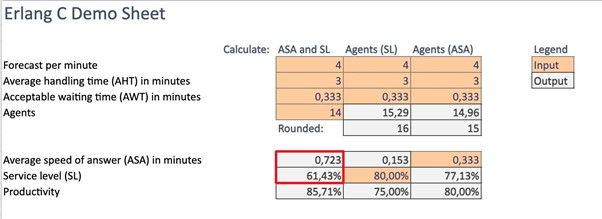The output of this computation can be visualized in the average speed of answer cell and the service level cell, which will be 0,723 min (43,38 seconds) and the service level will be 61,43%.

b) Calculation of number of agents on basis of service levels (SL)

To solve this task, the user must introduce the following parameters:

• Call arrivals per minute (Forecast-FC)
• Average Handling time in minutes (AHT)
• Acceptable Waiting Time in minutes (AWT)
• Service Level

The output of this computation can be visualized in the number of agents cell.

Example:

We would like to determine the required number of agents. We know that on average we have 240 calls per hour, the average handling time is 3 minutes, the acceptable waiting time is 20 seconds, and the service level is 80%.

The first step is to transform the given data into units that are expressed in minutes. The arrival rate should be transformed in minutes; in this case there will be (240/60=) 4 calls per minute and the acceptable waiting time will be (20/60=) 0,333 minutes.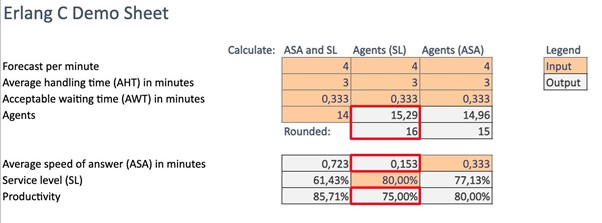Given the input data, the output of the software will be the need of 16 agents, the average speed of answer will be 0,153 minutes (9,18 seconds) and the productivity will be 75%.

c) Calculation of number of agents on basis of ASA

We would like to determine the number of agents. We know that on average we have 240 calls per hour, the average handling time is 3 minutes, the acceptable waiting time is 20 seconds, and the average speed of answer is 20 seconds.

The first step is to transform the given data into units that are expressed in minutes. The arrival rate should be transformed in minutes; in this case there will be (240/60=) 4 calls per minute, the acceptable waiting time will be (20/60=) 0,333 minutes, and the average speed of answer will be (20/60=) 0,333 minutes.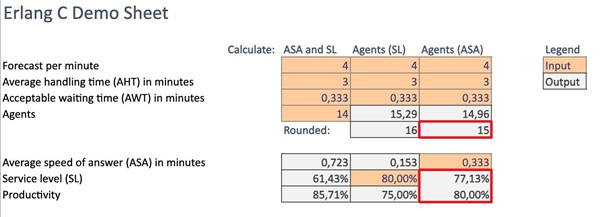The rounded output of this computation can be visualized in cell E8, which in this case will be 15 agents. The service level will be 77,13% and the productivity will be 80%.

Erlang X model is a model where the queued customers are impatient and abandon the queue after an exponentially distributed amount of time. Abandoned customers redial with a certain probability. This model can also take forecast errors into account. The Erlang model uses the following input parameters from the historical data:

• Call arrivals per minute (Forecast-FC)
• Average handling time in minutes (AHT)
• Average patience of calls in minutes
• Acceptable Waiting Time in minutes (AWT)
• Number of Agents
• Number of Lines

Within this Excel sheet, you can calculate three different scenarios using different input parameters:

a) Calculation of the average speed of answer and service level

In order to solve this task, the user must introduce the following parameters:

• Call arrivals
• Average Handling Time
• Acceptable Waiting Time
• Number of Agents
• Number of Lines

The output will include the following parameters:

• Service Level (in %)
• Abandonments (in %)
• Blocked Calls (in %)
• Productivity (in %)

Example:

We would like to determine the service level and the average speed of answer. We know that on average we have 240 calls per hour, the average handling time is 3 minutes, the average patience of callers is 1 minute, the acceptable waiting time is 20 seconds, the number of lines is 100, and the number of agents is 14.

The first step is to transform the given data into units that are expressed in minutes. The arrival rate should be transformed in minutes; in this case there will be (240/60=) 4 calls per minute and the acceptable waiting time will be (20/60=) 0,333 minutes.

The output will be the following: the average speed of answer is 0,1 minutes, the service level is 87,98%, the probability of abandonment is 0,0743, the probability of having any blocked calls is 0, while the calculated productivity is 79,34%.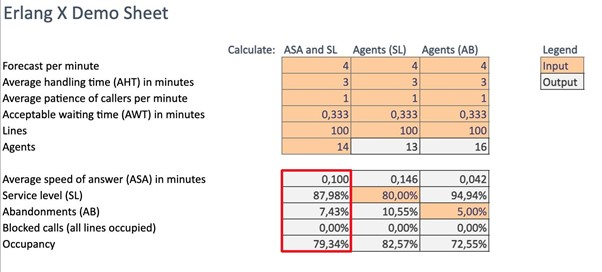b) Calculation of the number of agents on basis of the service level

In order to solve this task, the user must introduce the following parameters:

• Call arrivals
• Average Handling Time
• Average Patience of Calls
• Acceptable Waiting Time
• Number of Lines
• Service Level

The output will include the following parameters:

• Number of Agents
• Abandonments (in %)
• Blocked Calls (in %)
• Productivity (in %)

Example:

We would like to determine the required number of agents, given the fact we know that on average we have 240 calls per hour, the average handling time is 3 minutes, the average patience of callers is 1 minute, the acceptable waiting time is 20 seconds, the number of lines is 100, and the service level is 80% within 20 seconds.

The first step is to transform the given data into units that are expressed in minutes. The arrival rate should be transformed in minutes; in this case there will be (240/60=) 4 calls per minute and the acceptable waiting time will be (20/60=) 0,333 minutes.

The output will be the following: the average speed of answer is 0,146 minutes (8,76 seconds), the number of required agents is 13, the probability of abandonment is 0,1055, and the probability of having any blocked calls is 0, while the calculated productivity is 82,57%.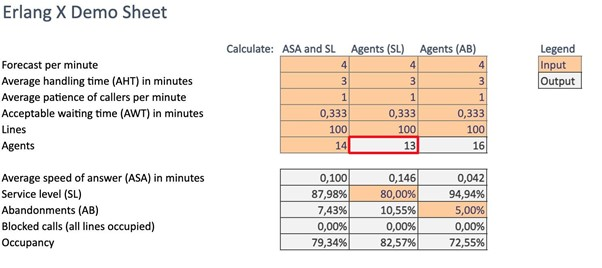c) Calculation of the number of agents on basis of abandoned calls

To solve this task, the user must introduce the following parameters:

• Call arrivals
• Average Handling Time
• Average Patience of Calls
• Acceptable Waiting Time
• Abandonments
• Number of Lines

The output will include the following parameters:

• Number of Agents
• Service Level (in %)
• Blocked Calls (in %)
• Productivity (in %)

Example:

We would like to determine the required number of agents given that we know that on average we have 240 calls per hour, the average handling time is 3 minutes, the average patience of callers is 1 minute, the acceptable waiting time is 20 seconds, the number of lines is 100, and the abandonments percentage is 5%.

The first step is to transform the given data into units that are expressed in minutes. The arrival rate should be transformed in minutes; in this case there will be (240/60=) 4 calls per minute and the acceptable waiting time will be (20/60=) 0,333 minutes.

The output will be the following: the number of agents is 16, the average speed of answer is 0,042 minutes (2,52 seconds), the service level is 94,54%, the probability of having any blocked calls is 0, while the calculated productivity is 72,55%.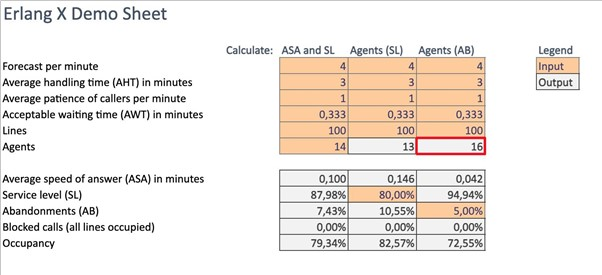The Erlang Chat model is like the Erlang X model. The distinguishing feature is that agents can now handle multiple chats at the same time. Handling multiple chats in parallel is often more efficient than handling each chat individually, one after the other. On the other hand, the time spent on a certain chat could increase when working on multiple chats at the same time. The Erlang model uses the following input parameters from the historical data:

• Chat arrivals per minute (Forecast-FC)
• Average handling time with 1 parallel chat in minutes (AHT)
• Average handling time with 2 parallel chat in minutes (AHT)
• Average handling time with 3 parallel chat in minutes (AHT)
• Average patience of chats in minutes
• Acceptable Waiting Time in minutes (AWT)
• Number of Agents
• Number of Lines

As an example, suppose it takes on average 12 minutes to handle a single chat. When working on 2 chats at the same time, this average handling time could increase to 14 minutes. And when handling 3 chats at the same time, the average handling time per chat could increase to 16 minutes. So, although the average handling time increases, it is still more efficient to do 3 chats in parallel.

The feature of different AHTs when doing a different number of chats in parallel can be entered in the Erlang Chat model by the AHT parameter. In this case, the AHT must be a range of values (instead of a single number as in the other models). The length of the range automatically determines the maximum number of chats that can be handled in parallel. Continuing from the example above, suppose that the maximum number of parallel chats is 3, and that the cells C5, C6, and C7 contain the values 12, 14, and 16 respectively. The AHT parameter must then be entered as the range C5:C7.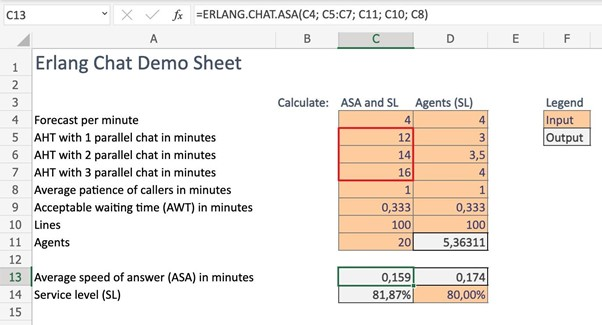## Miscellaneous

Please make sure you have a Microsoft 365 license with at least the following versions:
• Windows - Version 16.0.12527 or up
• Mac - Version 16.34 or up
• Web - Version May 2019 or up

The Erlang add-in provides Erlang functions in an Erlang C, X, Chat and Blending model. All functions and parameters can be found in the manual.

Yes, all parameters must be given in the same time unit. An example:

We would like to determine the service level and the average speed of answer in minutes, in the Erlang C demo sheet. We know that on average we have 240 calls per hour, the average handling time is 3 minutes, the acceptable waiting time is 20 seconds, and the number of agents is 14. The first step is to transform the given data into units that are expressed in minutes. The rates should be transformed in minutes; in this case there will be (240/60=) 4 calls per minute and the acceptable waiting time will be (20/60=) 0,333 minutes.

No, some parameters in the Erlang X functions are optional, these parameters are cursive and grey. Please note that when you leave an optional parameter empty, all remaining optional parameters must also be empty.

In the blending model, agents are able to work on different types of calls: inbound as well as outbound. It is assumed that there is an infinite amount of outbound calls to be done. When an agent finishes a call, they will always take the longest waiting inbound call from the queue, if there is any. In case the queue with inbound calls is empty, then a decision has to be made whether the agent takes an outbound call, or remains idle so that they are immediately available for the next arriving inbound call.

This decision is modeled by means of a threshold. Only when the threshold number of agents are left idle, then an agent who also becomes available is allowed to take an outbound call. For example, suppose there are 12 agents scheduled and the threshold is set to 3. This means that an outbound call can only be started when the fourth agent becomes idle (because then 3 agents are still left idle). Note that this does not mean that 3 agents are always idle. They will still work on inbound calls as soon as new inbound calls arrive.

The output of the blending model consists of the standard performance measures like SL, ASA, and productivity. Next to these, it also shows the average number of outbound calls that can be made.

The Definition parameter is an optional parameter that can be used to control how the service level is calculated. This parameter is only relevant when there are abandoned calls, so when the patience is also an input parameter. This parameter can be one of three values:

• 0: the virtual service level
• 1: the service level based on the answered calls
• 2: the service level based on the offered calls

We will illustrate this by an example. Suppose 10 customers have called, 8 out of those 10 are answered, and 2 out of 10 have abandoned. Furthermore, 7 out of the 8 answered calls are answered within the acceptable waiting time. Then the SL will be 7/8 according to Definition 1. On the other hand, the SL will only be 7/10 according to Definition 2.

Definition 0 is a little bit more advanced. It not only looks at the waiting time of answered calls, but also looks at the waiting time of abandoned calls. More specifically, it calculates the time that abandoned calls would have waited until service if they did not abandon. This is called the virtual waiting time. It then simply checks whether the virtual waiting time is less than the AWT for all offered calls.